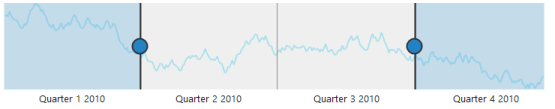# Populate Data

24 Nov 20174 minutes to read

When you provide data to RangeNavigator, it produces limited set of data. You can populate the RangeNavigator with data using the DataSource and Series properties.

## Add series to the RangeNavigator

The `Series` property provides access to a collection of all series that are defined explicitly within a RangeNavigator control. Each series is assigned with `Type` and name. It contains collection of data point, each point contains x value and y values. You can add data points to the series through `DataSource` property by providing field name to get the values from the DataSource in `xName` and `yName` options.

Animation can be enabled by setting `EnableAnimation` property as true and the series color can be customized by using `Fill` property in series.

• HTML
• ``````<ej:RangeNavigator ID="RangeNavigator1" runat="server" onClientSideLoad="loadingData">

<Series>

<ej:Series Name="ProductA" EnableAnimation="false" Type="Line" Opacity="0.5" Fill="#69D2E7"></ej:Series>

</Series>

<%--Code --%>

</ej:RangeNavigator>``````
• JS
• ``````<script type="text/javascript">

data = GetData();

sender.model.series.dataSource = data.Open;

sender.model.series.xName =  "XValue",

sender.model.series.yName = "YValue";

}

// Method to get data in JSON format

function GetData() {

var series1 = [];

var series2 = [];

var value = 100;

var value1 = 120;

for (var i = 1; i < 730; i++) {

if (Math.random() > .5) {

value += Math.random();

value1 += Math.random();

} else {

value -= Math.random();

value1 -= Math.random();

}

var point1 = { XValue: new Date(2010, 0, i), YValue: value };

var point2 = { XValue: new Date(2010, 0, i), YValue: value1 };

series1.push(point1);

series2.push(point2);

}

data = { Open: series1, Close: series2 };

return data;

}

</script>``````

The following screenshot illustrates the RangeNavigator that is populated with data using DataSource property in series.RangeNavigator that is populated with data using dataSource property in series